Mathematics

# LCM ( Lowest Common Multiple)

738 views

 1 Introduction 2 Definition 3 Methods to find LCM 4 Properties of LCM 5 Solved Examples 6 Practice Problems 7 Summary 8 Frequently Asked Questions (FAQs)

17 September 2020

## Introduction

The LCM or Lowest Common Multiple is essential in solving the addition, and subtraction of simple fractions where denominators are different. By using the LCM we can make the Denominators the same which will make the whole process very easy.

In real life, LCM is used to solve the problems related to Racetracks or Traffic lights, etc.

In this article, we will discuss the method of finding LCM, The properties of the LCM.

Before discussing the Lowest Common Multiple, we need to know, What is Multiple?

In mathematics, Multiples are the products of Natural Numbers.

When a and b both are Natural Numbers and b is a multiple of a then a is called a divisor or factor of b.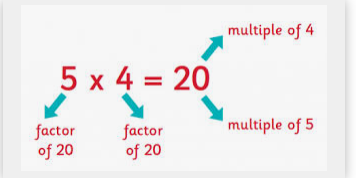## Definition of Lowest Common Multiple

The lowest common multiple or Least common multiple is also known as Least common divisor. It is the method of finding the least common multiples of a given set of Natural numbers.

For two or more given sets of Natural numbers, LCM is the smallest number that is evenly divisible by all the given natural numbers in the set.

For example, a given set of two Natural Numbers $$6$$ and $$10$$, the multiples $$6$$ and $$10$$ are ,

$$6=6,12,18,24, 30,42,48,54,60...$$

$$10=10,20,30,40,50,60…..$$

In this set $$6$$ and $$10$$ have many common multiples but the Lowest common multiple will be the smallest common multiple, that is $$30$$.

So, we can say that the LCM $$(6,10) = 30.$$

Along with the Lowest common multiple, one more important thing is there we need to know about, that is HCF or Highest common factor which is also known as GCF or Greatest Common factor.

In Mathematics, the greatest common factor or the highest common factor of two or more Natural numbers is the largest number that can divide each of the numbers of the given set.

For example, the greatest common factor of $$8,10,$$ and $$12$$ will be $$2$$ as all the $$3$$ numbers are divided by $$2$$ which is the highest common number. HCF and LCM are the two crucial parts of Mathematics.

## Methods to find The Lowest common Factor

There are five different methods to find the LCM of a given set of Natural numbers, They are

1. Listing multiples

2. Prime Factorisation

4. Division Method

5. Using the Greatest Common Factor or GCF

### 1. Finding LCM By Listing Multiples

• We have to find the multiples of each given number until we get the least multiples on all the lists.

• Identify the smallest number that is present on all of the lists.

• This smallest number is the LCM.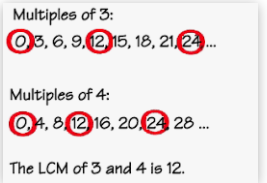### 2. Finding LCM by Prime Factorisation.

• We have to find all the prime factors of each number of a given set of Natural Numbers.

• Have to make a list of all the prime numbers found, as many times they occur.

• We have to multiply the prime factors to get the LCM. We can use the same process to find the LCM of more than $$2$$ Natural Numbers.

To find the LCM we can use Prime Factorisation using Exponents.

• List all the prime numbers by using the highest exponents for each factor.

• We have to Multiply the list of prime factors with exponents together to find the LCM.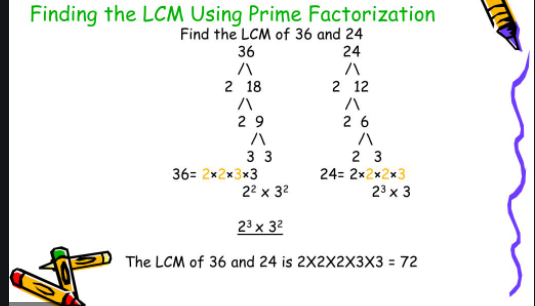### 3. LCM by using Cake Methods or Ladder Methods

The cake method or Ladder method is the method to find the LCM of a Natural Numbers by using division. It is the easiest and fastest way to find LCM.

• At first, we have to write the given numbers one beside another. Here $$50$$ and $$90.$$

• Now have to draw a layer of cake kind of structure around them.

• Then we have to take out anyone prime number which can divide all the given numbers and does not leave any reminder that is $$2.$$

• Now divide all the numbers by $$2$$ and write the quotient $$25$$ and $$45$$ below the original numbers.

• And then will draw another layer of cake around them.

• We have to repeat the process until we can’t divide both the numbers by the same number. Here it’s $$5$$ and $$9.$$

• To find the LCM we have to multiply all the numbers within the big $$\rm{L}$$

• LCM $$(50,90)=2\times5\times5\times9= 450$$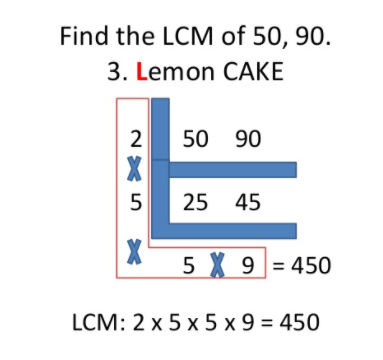### 4. LCM by using the Division Methods

In this method, we have to divide the given set of natural numbers by a prime number and have to continue to do so till the remainders will be prime numbers.

LCM will be the products of all the divisors and remaining prime numbers.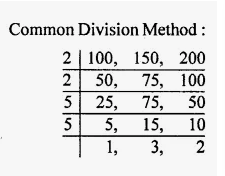### 5. LCM by GCF or The Greatest common factor

GCF or Greatest common factor is the common factor that can divide two or more natural numbers.

The formula by which we can find the LCM of a given set of natural numbers is,

 $$LCM (a,b)= \frac{(axb)}{GCF(a,b)}$$

\begin{align}&\text { Find L C M }(21,14,38) \\\\&\text{GCF }(14,38)=2 \\&\text{LCM }(14,38)=\frac{38 \times 14}{2}=266 \\&\text{GCF }(266,21)=7 \\&\text{LCM }(266,21)=\frac{266 \times 21}{7}=798 \\&\text{LCM }(21,14,38)=798\end{align}

### Formula to find LCM of a given set of natural numbers.

The LCM or The Lowest Common Multiple of $$2$$ natural numbers a and b is denoted as LCM(a,b).

The formulae to find the LCM (a,b) are,

 L.C. M formula for any two numbers $$\text{L. C. M}=\frac{a\times b}{gbc (a,b)}$$ L.C M formula for Fractions $$\text{L.C.M}=\frac{\text{L.C.M of Numbers}}{\text{H.C.F of Denominator}}$$

## Properties of LCM

1. LCM is Associative that means, $$\text{LCM(a,b)= LCM(b,a)}$$

2. LCM is distributive, that means $$\text{LCM(da, db, dc) = dLCM(a, b, c)}$$

3. LCM is Communicative, that means

$$\text{LCM (a, b, c) = LCM (LCM (a, b), c) = LCM (a, LCM(b, c)).}$$

4. The LCM of $$2$$ or more numbers can not be less than the smallest numbers given in the set. For example, LCM $$(6,8)$$ can not be less than $$6$$ and $$8.$$

5. In a given set of two natural numbers if one number is the factor of the other number, then the LCM will be the bigger number.

Example, $$\text{LCM }(9,27) = 27$$ as $$9$$ is a factor of $$27.$$

6. The LCM of $$2$$ prime numbers will be the product of the two given numbers. Example, $$\text{LCM } (5,7)=35.$$

## Some examples of solved LCM problems are: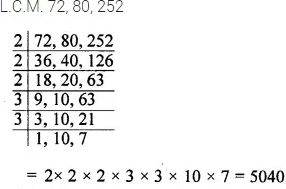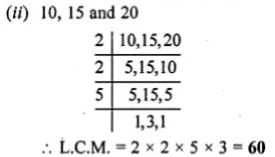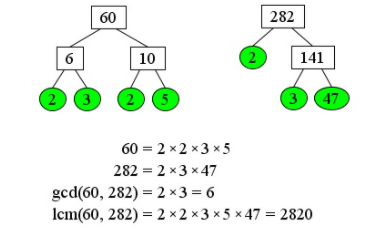Examples of LCM of fractions

 $$\text{L.C.M}=\frac{\text{LCM of the Numerators}}{\text{HCF of the Denominators}}$$

Let us see this using examples of $$\frac{2}{9}$$ and $$\frac{8}{21}.$$

1. LCM of $$9$$ and $$21 = 63,$$

2. Now multiply first fraction by $$\frac{63}{9} = 7$$

Multiply second fraction by $$\frac{63}{21} = 3$$

1st fractions is $$=\frac{(2 x 7)}{(9 x 7)} = \frac{14}{63}$$

and 2nd fraction is$$= \frac{(8 x 3)}{(21 x 3)}= \frac{24}{63}$$

3. Now both denominators are same so LCM of numerators will be$$14$$ and $$24 = 168.$$

4. Hence LCM of $$\frac{2}{9}$$ and $$\frac{6}{21}$$ is $$(\frac{168}{63})$$

After simplification $$\frac{168}{63} = \frac{8}{3}$$

### Program to find the GCD of two integers:

 int gcd(int A, int B) { if(A==0 || B==0)                 return max(A,B);                 if (A%B ==0) return B;                 if (A < B) swap(A, B);                 while (B > 0)                 {                                A = A%B;                                swap(A, B);                 }                 return A; }

### Program to find LCM of two integers:

 int lcm(int A,int B) {                 return (A*B)/gcd(A,B); }

## Practice problems of LCM

Q.1.  What is the L.C.M. of $$25, 30, 35,$$ and $$40$$?

Q.2. Find the L.C.M. of the given numbers by division method.

(i) $$20$$ and $$44$$

(ii) $$36, 24$$ and $$40$$

(iii) $$45$$ and $$120$$

Q.3.  Find the lowest number which leaves $$4$$ as the remainder when divide by $$9$$ and $$12.$$

Q.4.  The product of the L.C.M. and H.C.F. of two numbers is $$80.$$ If one of the numbers is $$20,$$ what will be the other number?

## Summary

So far we have learnt about Multiples, Lowest common multiples of two numbers, and also introduced the topic of Greatest common divisor. LCM and GCD are important aspects of any set of numbers and tell a lot of information about their behaviour in basic addition, subtraction, division etc. LCM helps in solving a variety of problems both in Mathematics and in everything that needs Mathematics to solve its problems.

Written by Snigdha Jha, Cuemath Teacher

## What is LCM of two numbers?

LCM of two numbers is defined as the least common multiple of those two numbers i.e. the smallest number that is divisible by both the given numbers. From the definition it is clear that

$$\text{min(A,B) <= LCM(A,B) <= A*B}$$

## What is the GCD of two numbers?

GCD of two numbers is defined as the Greatest Common Divisior of those two numbers i.e. the biggest number that can divide both the given numbers.

$$\text{1 <= GCD(A,B) <= min(A,B)}$$

## What is the relationship between LCM and GCD of two numbers?

The product of the LCM and GCD of two numbers is indeed the product of the two numbers.

$$\text{LCM(A,B) * GCD(A,B) = A*B}$$

## What are co-prime Numbers?

Co-Prime Numbers are those that have their $$\text{GCD=1.}$$

GIVE YOUR CHILD THE CUEMATH EDGE
Access Personalised Math learning through interactive worksheets, gamified concepts and grade-wise courses